Courses

# Properties of Fluids & Turbulent Flow in Pipes - MCQ Test 2

## 30 Questions MCQ Test GATE Mechanical (ME) 2022 Mock Test Series | Properties of Fluids & Turbulent Flow in Pipes - MCQ Test 2

Description
This mock test of Properties of Fluids & Turbulent Flow in Pipes - MCQ Test 2 for Mechanical Engineering helps you for every Mechanical Engineering entrance exam. This contains 30 Multiple Choice Questions for Mechanical Engineering Properties of Fluids & Turbulent Flow in Pipes - MCQ Test 2 (mcq) to study with solutions a complete question bank. The solved questions answers in this Properties of Fluids & Turbulent Flow in Pipes - MCQ Test 2 quiz give you a good mix of easy questions and tough questions. Mechanical Engineering students definitely take this Properties of Fluids & Turbulent Flow in Pipes - MCQ Test 2 exercise for a better result in the exam. You can find other Properties of Fluids & Turbulent Flow in Pipes - MCQ Test 2 extra questions, long questions & short questions for Mechanical Engineering on EduRev as well by searching above.
QUESTION: 1

Solution:
QUESTION: 2

Solution:

Ans. (d)

QUESTION: 3

### Assertion (A): In a fluid, the rate of deformation is far moreimportant than the total deformation itself. Reason (R): Afluid continues to deform so long as the external forces areapplied.

Solution:

Ans. (a) Both A and R correct and R is correct explanation for A

QUESTION: 4

Newton’s law of viscosity depends upon the

Solution:

Ans. (c) Newton's law of viscosity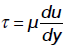where, ζ→ Shear stress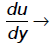Rate of strain

QUESTION: 5

Consider the following statements:
1. The friction factor in laminar flow through pipes is independent of roughness.
2. The friction factor for laminar flow through pipes is directlyproportional to Reynolds number.
3. In fully turbulent flow, through pipes, friction factor is independentof Reynolds number.Which of the statements given above are correct?

Solution:

Ans. (b) The friction factor in laminar flow through pipes is given by the expression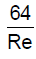which is independent of roughness.
The friction factor for laminar flow through pipes is inversely proportional to Reynolds number.
In fully turbulent flow through pipes friction factor is independent of Reynolds number.

QUESTION: 6

In a fully turbulent flow through a rough pipe, the friction factor 'f' is(Re is the Reynolds number and ξS / D is relative roughness)

Solution:

Ans. (b)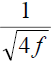= 2log10 ( R / K ) + 1.74;
f is independent of Reynolds number and
depends only on relative roughness (k/D). This formula is widely used. But experimental facts reveals that the friction factor 'f' is also depends on Reynolds
number. As many modern handbook uses empirical formula with Reynold’s number our answer will be (b).

QUESTION: 7

What are the dimensions of kinematic viscosity of a fluid?

Solution:

Ans. (b)

QUESTION: 8

Decrease in temperature, in general, results in

Solution:

Ans. (c) The viscosity of water with increase in temperature decreases and that of air increases.

QUESTION: 9

Match List-I (Type of fluid) with List-II (Variation of shear stress) and select the correct answer: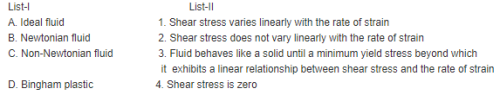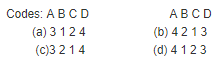Solution:

Ans. (d)

QUESTION: 10

Assertion (A): Blood is a Newtonian fluid. Reason (R): The rate of strain varies non-linearly with shearstress for blood.

Solution:

Ans. (d) A is false but R is true.

QUESTION: 11

In an experiment, the following shear stress - time rate of shearstrain values are obtained for a fluid:
Time rate of shear strain (1/s):       0     2       3     4
Shear stress (kPa):                        0    1.4    2.6   4

Solution:

Ans. (d)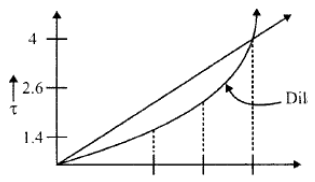QUESTION: 12

Surface tension is due to

Solution:

Ans. (b) Surface tension is due to cohesion between liquid particles at the surface, where as capillarity is due to both cohesion and adhesion. The property of cohesion enables a liquid to resist tensile stress, while adhesion enables it to stick to another body.

QUESTION: 13

What is the pressure inside a soap bubble, over the atmospheric pressure if its diameter is 2 cm and the surface tension is 0·1N/m?

Solution:

Ans. (c)

QUESTION: 14

Which one of the following is correct? The capillary rise on depression in a small diameter tube is

Solution:

Ans. (c) The capillary rise on depression is given by,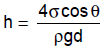QUESTION: 15

Assertion (A): A narrow glass tube when immersed into mercurycauses capillary depression, and when immersed into watercauses capillary rise.
Reason (R): Mercury is denser than water.

Solution:

Ans. (b) Causes of capillary depression: Adhesion is less than cohesion, the wetting tendency is less and the angle of contact is high.

QUESTION: 16

The capillary rise at 200C in clean glass tube of 1 mm diameter containing water is approximately

Solution: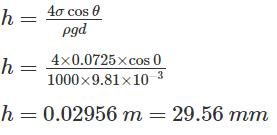For water and glass, Contact Angle (θ)=0º and Surface tension is 0.0725 N/m
For mercury and glass, Contact Angle (θ)=128º and Surface tension is 0.52 N/m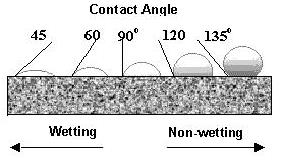QUESTION: 17

In a turbulent flow,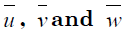are time average velocity components.The fluctuating components are u', v' and w’ respectively. Theturbulence is said to be isotropic if:

Solution:

Ans. (d) Isotropic Turbulence: Turbulence whose properties, especially statistical correlations, do not depend on direction.
Strictly speaking, isotropy, i.e.  ndependence of orientation, implies homogeneity, i.e. independence of position in space. In most situations, all the averaged properties of isotropic turbulence can also be assumed to be invariant under reflection in space.
For Isotropic turbulence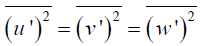and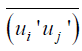Where i ≠ j

QUESTION: 18

If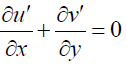for a turbulent flow then it signifies that

Solution:

Ans. (a) As it follow conserve continuity equations.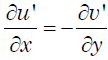It is postulated that if at an instant there is an increase in u' in the x-direction, it will be followed by an increase in v' in the negative y-direction. In other words,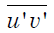is non-zero and negative.

QUESTION: 19

When we consider the momentum exchange between two adjacent layers in a turbulent flow, can it be postulated that if at an instantthere is an increase in u' in the x-direction it will be followed by achange in v' in the y direction?

Solution:

Ans. (c)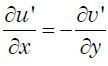It is postulated that if at an instant there is an increase in u' in the x-direction,
it will be followed by an increase in v' in the negative y-direction. In other
words,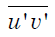is non-zero and negative.

QUESTION: 20

In fully-developed turbulent pipe flow, assuming 1/7th power law, the ratio of time mean velocity at the centre of the pipe to that averagev elocity of the flow is:

Solution:

Ans. (d) =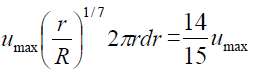or without calculating this we may say that it must be less than one and option (d) is only choice.

QUESTION: 21

A U-tube is made up of two capillaries of diameters 1.0 mm and 1.5 mm respectively. The U tube is kept vertically and partially filled with water of surface tension 0.0075kg/m and zero contact angles.
Calculate the difference in the level of the menisci caused by the capillarity.

Solution:
QUESTION: 22

A metal plate 1.25 m × 1.25 m × 6 mm thick and weighting 90 N is placed midway in the 24 mm gap between the two vertical plane surfaces. The Gap is filled with an oil of specific gravity 0.85 and dynamic viscosity 3.0N.s/m2. Determine the force required to lift the plate with a constant velocity of 0.15 m/s.

Solution:

Ans. 168.08N

QUESTION: 23

In order to form a stream of bubbles, air is introduced through a nozzle into a tank of water at 20°C. If the process requires 3.0 mm diameter bubbles to be formed, by how much the air pressure at the nozzle must exceed that of the surrounding water? What would be the absolute pressure inside the bubble if the surrounding water is at 100.3 kN/m2? (σ = 0.0735 N/m)

Solution:

Ans. Pabs= 100.398 kN/m2 (Hint. Bubble of air but surface tension of water).

QUESTION: 24

The volumetric change of the fluid caused by a resistance is known as

Solution:
QUESTION: 25

A 400 mm diameter shaft is rotating at 200 rpm in a bearing of length 120 mm. If the thickness of oil film is 1.5 mm and the dynamic viscosity of the oil is 0.7 Ns/m2 determine:
(i) Torque required overcoming friction in bearing;
(ii) Power utilization in overcoming viscous resistance;

Solution:
QUESTION: 26

Match List-I (Physical properties of fluid) with List-II (Dimensions/Definitions) and select the correct answer: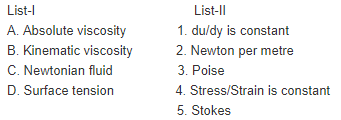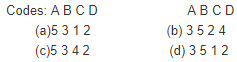Solution:

Ans. (d)

QUESTION: 27

Fluids that require a gradually increasing shear stress to maintain a constant strain rate are known as

Solution:

Ans. (a)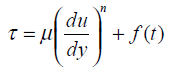where f(t) is increasing

QUESTION: 28

Consider the following statements:
1. Gases are considered incompressible when Mach number isless than 0.2
2. A Newtonian fluid is incompressible and non-viscous
3. An ideal fluid has negligible surface tension
Which of these statements is /are correct?

Solution:

Ans. (d)

QUESTION: 29

At the interface of a liquid and gas at rest, the pressure is:

Solution:

Ans. (a)

QUESTION: 30

Which one of the following sets of conditions clearly apply to anideal fluid?

Solution:

Ans. (b)